# The price

The price of the land increased by 17%. What was the original price of the land if it now costs 46800 €?

x =  40000 Eur

### Step-by-step explanation:

x • (1 + 17/100) = 46800

117x = 4680000

x = 40000

Our simple equation calculator calculates it.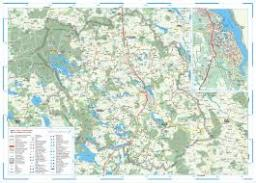Did you find an error or inaccuracy? Feel free to write us. Thank you!Tips to related online calculators
Do you have a linear equation or system of equations and looking for its solution? Or do you have a quadratic equation?

## Related math problems and questions:

• Sale offThe TV went down 10% and then 10% off the original price again. Now it costs 300 €. What was its original price?
• BookshelveBookshelve with an original price of € 200 twice become cheaper. After the second discounted by 15% the price was € 149.60. Determine how much % become cheaper for the first time.
• Double price reduction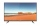The price of TV has been reduced twice. First by 15% and later by another 10% of the reduced price. After this double price reduction, the TV was sold for 8,874 crowns. What was the original price of the TV?
• The notebook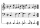After rising by 40%, the notebook cost 10.50 euros. How much did this notebook cost if it increased in price by only 20% instead of 40%?
• Former priceThe price of an article is cut by 10%, to restore it to the former value, by what percent the new price must be increased?
• Final priceThe book with the original price of CZK 850 was reduced by 20%. In a week, it was raised by 20% of the new price. Find the final price of the book. How much percents of the original price of the book is the final price?
• Product V2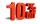The price of product was increased by 12%. Price increased by 273. How much money it would cost this product, if it was on the contrary by 19% discounted.
• Discount and pricesAfter a 29% discount, the new product price was € 426. What was the original price of the product?
• DiscountLadies sweater was twice discounted. First by 8%, then by 8% of the new price. Its final price was 66 €. Determine the original price of the sweater.
• Ice skates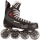Ice skates were raised twice, the first time by 25%, the second time by 10%. After the second price, their cost was 82.5 euros. What was the original price of skates?
• Sale goods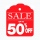Sale goods were reduced twice. First by 15% and then by another 20% of the new price. What percentage of the original price was the final price?
• Price saleoffShoes standing y euros. At first, they were discounted by 12% and then 50% of the new amount. After this double cheapening the cost was exactly 22 euros. Determine the original price of the shoes.
• The ballThe ball was discounted by 10 percent and then again by 30 percent. How many percent of the original price is now?
• Prices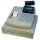The price of the product was increased by 35%. How many percents of the new price we have to make it cheaper so that its price is equal to the original price?
• The TV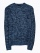The TV costs CZK 9,999. First, they reduced her price by 15%, then they became more expensive by 15%. How much does television cost now?
• Tennis racketTennis racket became cheaper by 16% in winter. In the spring, the price increased by 15%. After this increase, it cost € 38. How much did the rocket cost in the winter before cheapening?
• Price increase 2xIf two consecutive times we increase the price of the product by 20%, how many % is higher final price than the original?